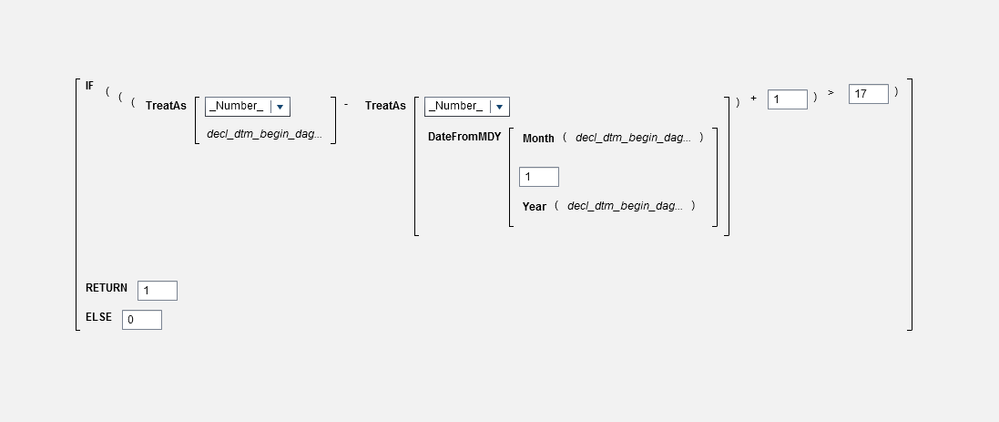## Calculate number of weekdays between two days

Hi all,

I am looking for a way to find the number of weekdays between the first day of the month and a given date.

I've calculated the number of days like in the screenshot, but I can't find a way to take only weekdays into account.I know I can find the day of the week via the DayOfWeek function, but couldn't find a way to incorporate this in the formula above.

Basically I need to see the number of weekdays elapsed on a given date compared to the start of that month.

I'd appreciate it if anyone could help me with this.

Bas

1 ACCEPTED SOLUTION

Accepted Solutions

## Re: Calculate number of weekdays between two days

You can use SAS code in VA 7.4, but only in SAS Studio, the web-basing coding interface packaged with VA. I think it would be easier to add this as a new column to your data before loading into VA LASR memory using @Reeza's approach.

6 REPLIES 6

## Re: Calculate number of weekdays between two days

Are you looking for a Point&Click answer in VA or a simple code answer?

## Re: Calculate number of weekdays between two days

One or the other. That doesn't really matter. I am somewhat familiar with the coding used in VA.

I have only got access to VA 7.4, so if it works there, that would be great.

## Re: Calculate number of weekdays between two days

The INTNX function is available in CAS.

In Base SAS that would be:

``intnx('weekday', date1, date2)``

## Re: Calculate number of weekdays between two days

You can use SAS code in VA 7.4, but only in SAS Studio, the web-basing coding interface packaged with VA. I think it would be easier to add this as a new column to your data before loading into VA LASR memory using @Reeza's approach.

## Re: Calculate number of weekdays between two days

Thank you all for your replies. I think the suggestion given by SASKiwi should be the easiest way for us.

We'll add the extra column to the data, so we can use it for our calculations instead of trying to build it all in the VA interface.

Thanks!

## Re: Calculate number of weekdays between two days

Hi @Basl,

I'm glad you found some useful info! If @SASKiwi's reply was the exact solution to your problem, can you "Accept it as a solution"? Or if it was particularly helpful, feel free to "Like" it. This will help other community members who may run into the same issue know what worked.

Thanks!
Anna